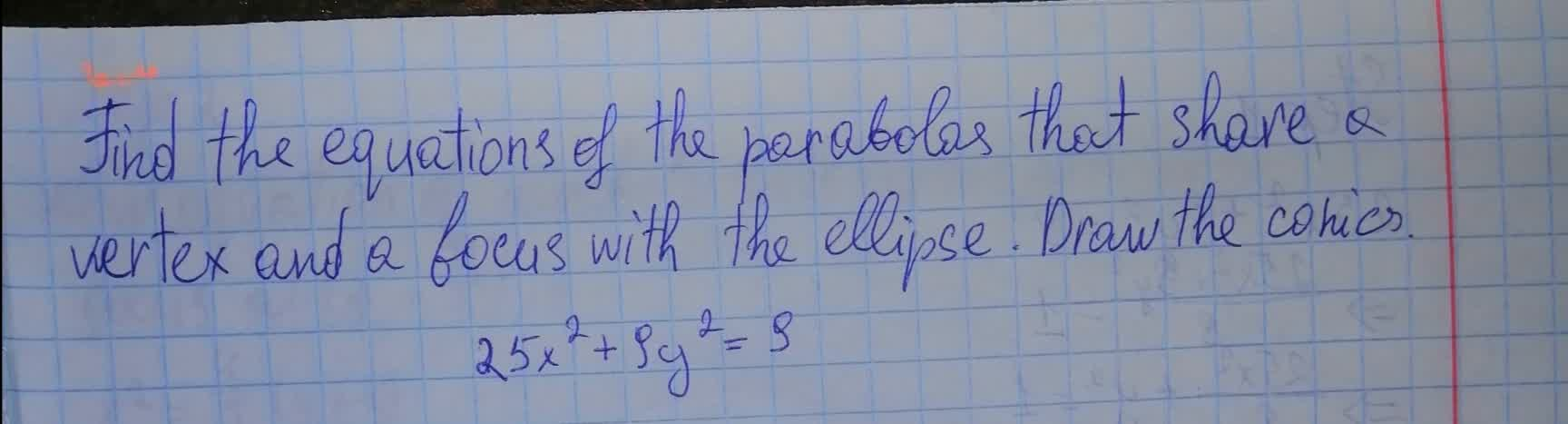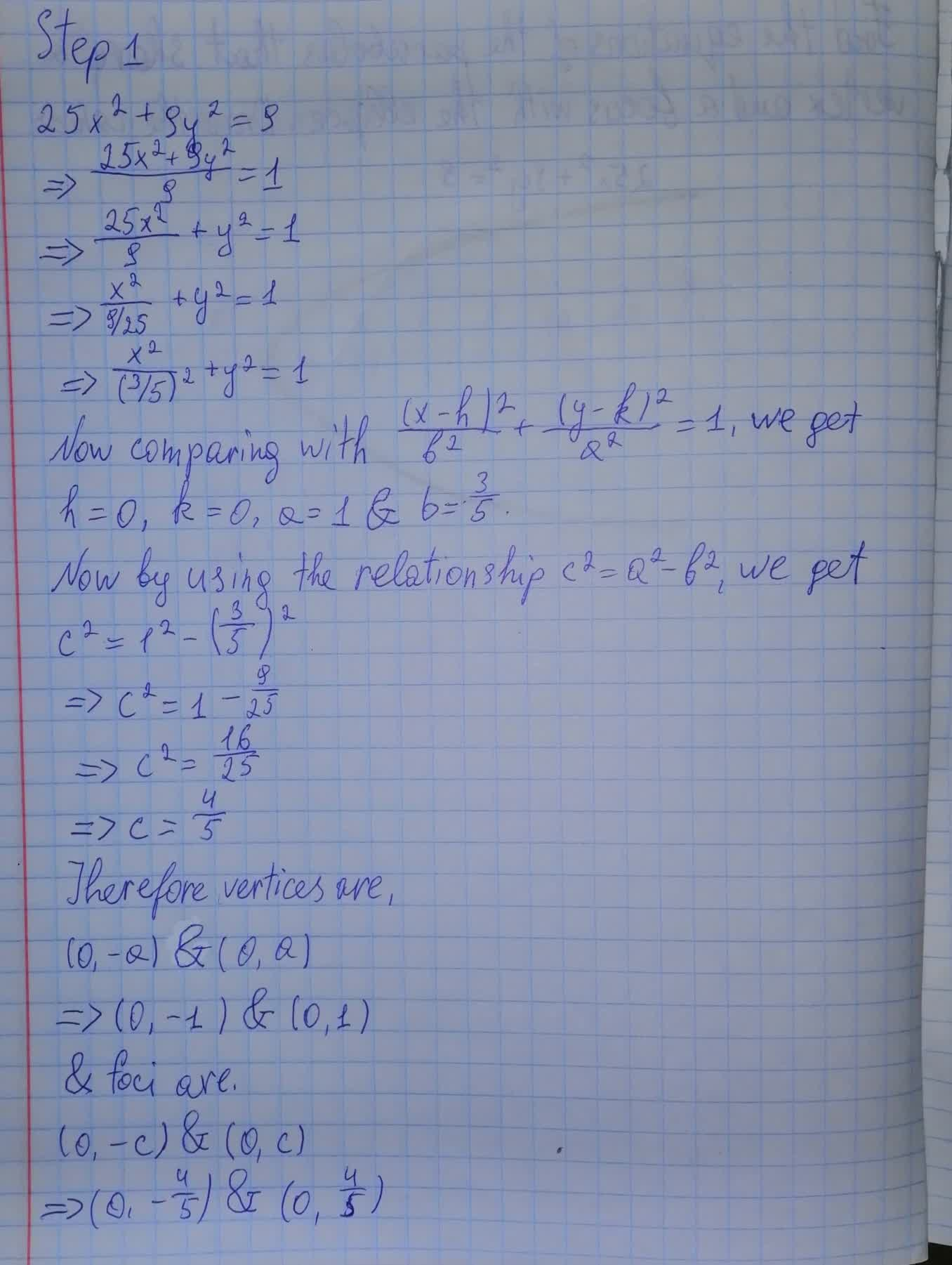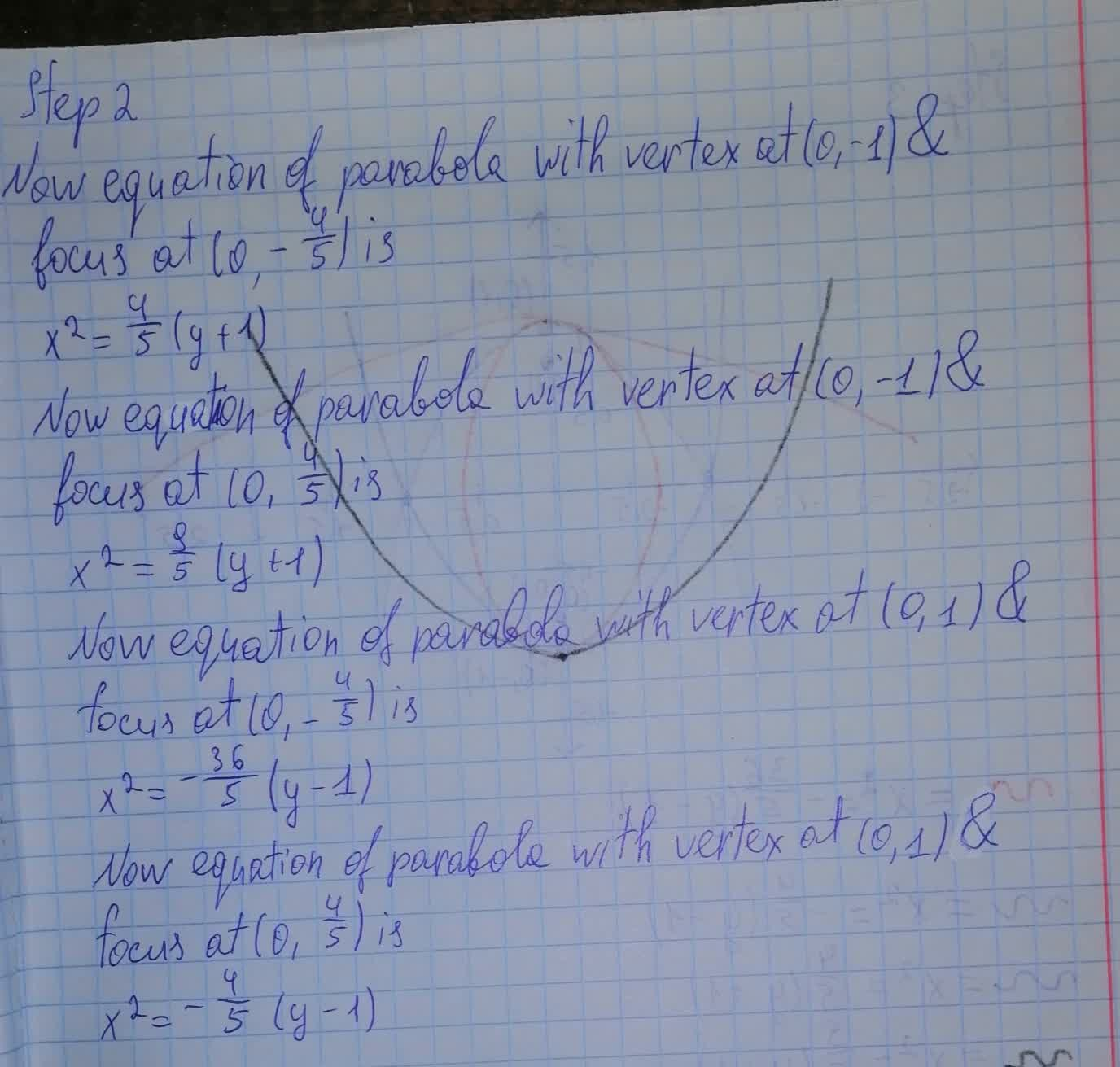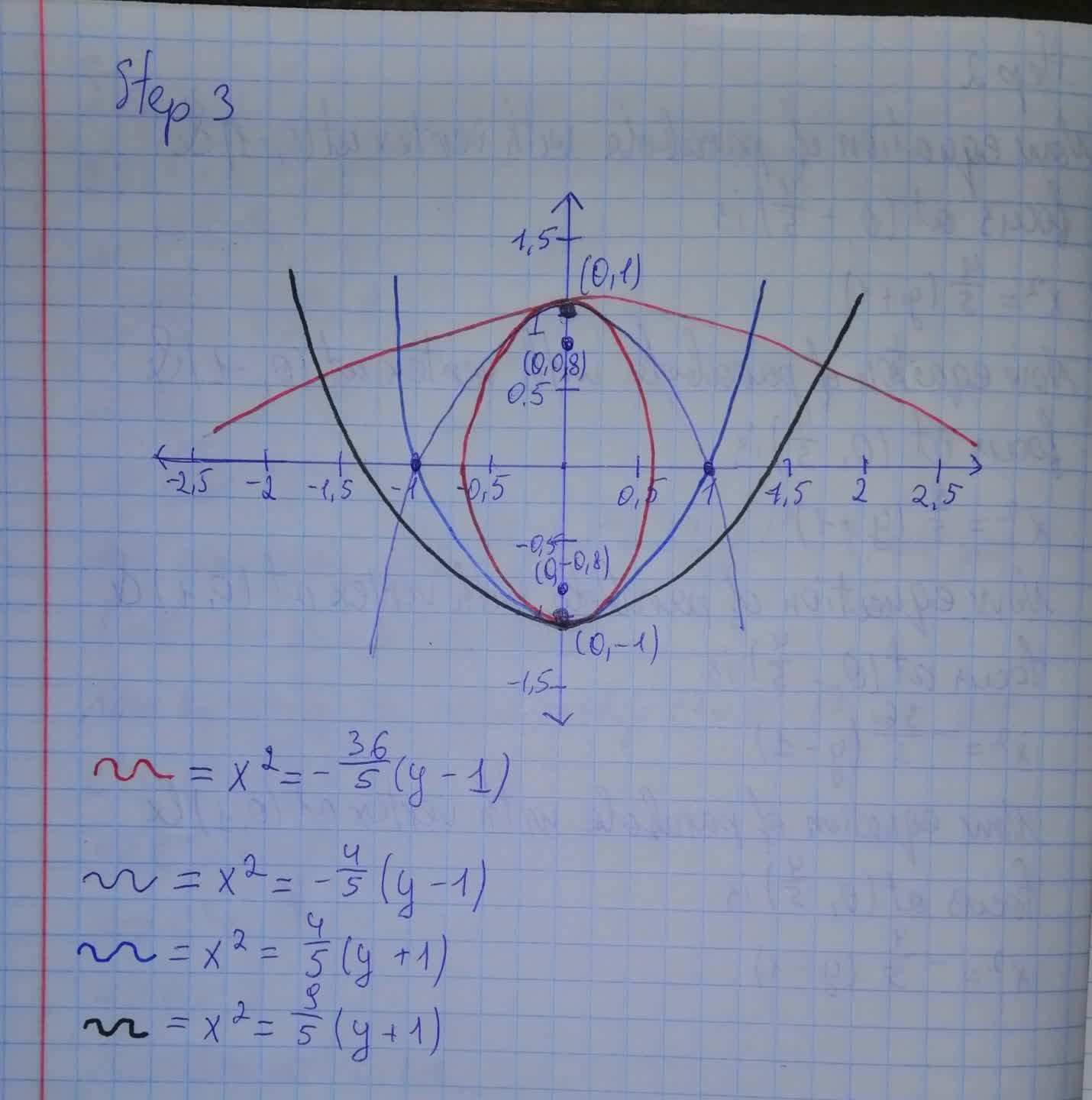Question# Find the equations of the parabolas that share a vertex and a focus with the ellipse. Draw the conics. 25x^2 + 9y^2 = 9

Conic sections
ANSWEREDFind the equations of the parabolas that share a vertex and a focus with the ellipse. Draw the conics. $$\displaystyle{25}{x}^{{2}}+{9}{y}^{{2}}={9}$$